American Journal of Modern Physics
Volume 4, Issue 4, July 2015, Pages: 212-216

Breaking of Charge Symmetry in Quantum Mechanics

Klaus Lewin

Sektion Physik, Humboldt-University, Berlin, Germany (retired since 1992)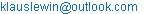Klaus Lewin. Breaking of Charge Symmetry in Quantum Mechanics. American Journal of Modern Physics. Vol. 4, No. 4, 2015, pp. 212-216. doi: 10.11648/j.ajmp.20150404.18

Abstract: Deuteron photo-decay with subsequent proton detection represents an experiment similar to Bohm’s version of the EPR arrangement replacing the entangled spin singlet state of the two-fermion system by entangled isospin states. Dropping the conception of truth, the detection as charge symmetry breaking event a posteriori leads up to a pre-existing proton identifying the second possibly far apart nucleon as neutron. That explains what the entangled isospin state predicts without assumption of a superluminal correlation.

Keywords: Deuteron Photo-Decay, Entangled Isospin States, Charge Symmetry, Proton Detection, Retroactivity

1. Introduction

As well-known, quantum mechanics (QM) represents one of the most successful physical theories predicting experimental results. As counterpart to classical mechanics it also should be able to describe microphysical dynamical processes occurring independently of human observers and it should be free of paradoxes and artificial assumptions from outside its mathematical formalism. Concerning these problems the discussion – rooting historically in the famous Einstein-Bohr controversy – is still alive. Since the violation of Bell’s inequalities  by the results of so-called EPR-Bell experiments during the seventies starting with , local realism in the sense of the historical EPR paper  classifying quantum mechanics (QM) as incomplete theory had to be abandoned. Completeness of QM with fundamental meaning of the indeterminacy relations was the only alternative and seemed to favor the Copenhagen interpretation that was accepted by a majority. However, long-standing problems like the EPR paradox and the understanding of the wave function collapse remained. Moreover, the results of coincidence measurements in EPR-Bell experiments and of Bohm’s version of the EPR arrangement (EPRB)  couldn’t be understood without instantaneous or superluminal correlations between the two detections (nonlocality). That contradiction to special relativity induced a discussion about the correctness of the formalism of QM. In that context, P.A.M. Dirac remarked 1972: " Nonlocality is against the spirit of special relativity… . Certainly, one is not satisfied by such a theory. It seems to me that the problem of compatibility of quantum theory with special relativity is not yet solved" . An essential point of the discussion is the question of pre-existing quantum numbers before a particle detection mostly provoked by the thinking of experimentalists. Regarding neutron detections in neutron interferometry H. Rauch remarked: "The question of how the well-defined particle properties of the neutron are transferred through the interferometer is not a meaningful one in the Copenhagen interpretation, but from the physical point of view it should be an allowed one" . However, that interpretation a priori excludes the consideration of particle properties during wave function evolution because of its so-called conception of truth rejecting the discussion of quantum numbers that are not measurable directly (see, e.g. ). On the other hand, if one would be able to interpret QM as complete theory taking into account pre-existing quantum numbers, one could solve the collapse problem and the EPR paradox. A corresponding alternative to the Copenhagen interpretation was discussed by D. Mermin taking into account the following principles [8,9]: "QM represents a theory describing an objective reality independent of observers and their knowledge. Such a theory has to deal with individual systems because the world contains them. In a nondeterministic world, probability has nothing to do with incomplete knowledge and ought not to require an ensemble of systems for its interpretation". The assumption of an objective reality includes particle presence during wave packet evolution with indeterminate external dynamical parameters (see [10,11]). In the following we describe two EPR-type experiments favoring this view on the physical world. After a review of fundamental aspects in section 2 and of Bohm’s version of the EPR arrangement (EPRB) in section 3, we consider in the main section 4 deuteron photo-decay with subsequent proton detection representing a similar situation in which the entangled spin singlet state of EPRB is replaced by corresponding isospin states. In objective reality both these arrangements include quantum numbers pre-existing before detection as discussed in the following sections.

2. Symmetries and Quantum Mechanics

Following [10,11] we shortly sketch some aspects of the general group theoretical base of QM remembering to the close connection between symmetries and QM: Translational, rotational and time invariance lead to momentum, angular momentum and energy eigenvalue equations and to the commutation relations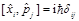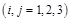(1)

[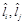]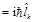(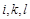cyclic)                      (2)

In usual notation. Together with the representation of dynamical variables by self-adjoined operators and with the Schroedinger equation in Hilbert space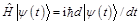(3)

the commutation relations (1, 2) represent the mathematical base of QM. The number of possible symmetry transformations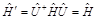(4)

is maximal for the free Hamiltonian and reduces after introduction of more or less asymmetric interaction terms including boundary conditions. The linearity of (3) and the absence of classical initial conditions lead to wave function solutions that are ruled by the Hamiltonian alone where eq.(4) is closely related to symmetries of the state. Non-zero standard deviations of dynamical variables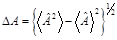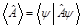(5)

and the absence of trajectories do not express a lack of knowledge but are related to symmetries/boundary conditions of the dynamical system.

Long-standing experience suggests that ordinary QM ("wave mechanics") is not able to solve problems around the particle structure of matter during wave function evolution including the wave function collapse which corresponds to the photoelectric effect that also cannot be explained in classical electrodynamics but needs the quantized theory (QED).

The essential point to explain the wave function collapse as symmetry breakdown is particle presence in the wave packet with indeterminate external dynamical parameters according to their standard deviations (5). That particle presence only can be accounted for by field quantization (see [10, 11]).

If no orbital angular momentum exists, (2) as expression of rotational invariance contains the spin components instead of total angular momentum, i.e.

[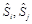] =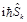(i, j, k  cyclic).              (6)

This case – represented by the unitary symmetry group SU(2) in spin space – has an analogy in the abstract isospin space (I-spin space) if one replaces rotational invariance by charge independence of strong interactions. In the special case of nuclear forces the spin up/spin down eigenstates correspond to the proton/neutron eigenstates whereas superposition states with indefinite spin value correspond to a nucleon with indefinite electric charge. Thus, (6) is valid also in isospin space if one replaces the spin components Si by I-spin components

[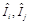]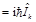(i, j, k  cyclic).                (6a)

3. Bohm’s Version of EPR and Elimination of the Paradox

Because of a remarkable analogy between the entanglement of spin and isospin states we review the situation in Bohm’s version of EPR . Following [10,11] we consider a compound C decaying at a time t0 into two identical fermions (C→C1+C2) with spin ½ forming a spin singlet state 1S0. Because of spherical symmetry of the 1S0 state, all three components of the spin vectors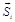(i = 1,2) of the two constituents are indeterminate under the condition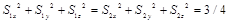(7)

which can be called hidden spin conservation because a direction of the two spin vectors is not defined. At some time t1, when a large macroscopic distance is reached between C1 and C2, a Stern-Gerlach (SG) device measures a spin component S1z of C1 in a given z direction determined by the orientation of the apparatus. Assume, the result is the spin eigenvalue s1z = +½ (detection of C1 at the lower part of the screen). One obtains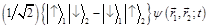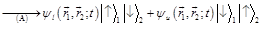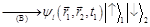(8)

where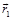and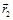are the coordinates of C1 and C2 , respectively.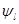and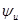represent the lower and the upper branch wave in the SG device for C1 . The first transition (A) in (8) describes the deflection by the inhomogeneous magnetic field not disturbing the unitary evolution whereas the second transition (B) describes the detection. The entangled state (8) predicts a definite opposite spin component of the second particle even in the case of a space-like distance between the two particles in the moment of detection ( Bohm’s version of the EPR paradox).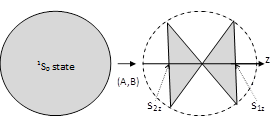Fig. 1. Situation before and after the SG detection of C1 in plane projection.

The spherical symmetry of the 1S0 state goes over into the more special symmetry of the lateral cone surfaces with definite spin eigenvalues s1z and s2z obeying the conservation law s1z + s2z = 0.

However, if the conception of truth is abandoned, it’s obvious a posteriori after the detection (B) that immediately before it a pre-existing fermion C1 with s1z = +½ was near the detection point in the lower channel (lower branch wave before detection) and can be traced back through that channel to the decay centre, i.e., this detection retroactively breaks the spin up – spin down symmetry of the two-branch wave function and consequently also the spherical symmetry of the 1S0 spin state reducing it to a cone symmetry with definite s1z = +½ (Fig. 1). Thus because of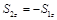, it’s evident after detection of C1 that the fermion C2 has left the decay centre with definite spin eigenvalue s2z = -½ compelling its spin also onto a cone surface as consequence of the retroactive effect of symmetry breaking by detection of C1. A delayed SG measurement at C2 in z direction at some time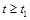with certainty would yield this result as predicted by the entangled state (8) although nothing in context with the measurement result at C1 did occur at that possibly far apart constituent.

4. Deuteron Photo-Decay and Breaking of Charge Symmetry by Proton Detection

Again we study the decay of a two-fermion bound S state into its constituents with subsequent detection of one decay fragment. Parallel to EPRB where the spin singlet state has a central role as entangled state, here the two isospin states of the neutron-proton system obtain a corresponding role.

The decay of a deuteron into two nucleons will be considered with subsequent proton detection that occurs on the inner surface of a hollow detection hemisphere around the decay center in the left semi-space whose radius R is large compared with the radius rc of the decay center: R>>rc (Fig. 2). We assume that only electrically charged particles can be detected and study the photo decay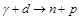of a deuteron into its nucleon constituents by absorption of a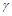quant whose energy minimally exceeds the deuteron binding energy of about 2.2 MeV. Because the latter is three orders of magnitude smaller than the nucleon mass, the photon momentum is negligible in comparison with the nucleon momenta after the decay, i.e. the nucleons approximately leave the decay center in opposite directions like in a spontaneous decay. Describing the free evolution of the two-nucleon system in the time interval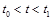between the decay and the detection, one can approximately use a one-particle state with reduced mass depending on the particle distance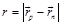and then express the evolution by an outgoing spherical wave function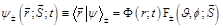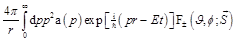(9)

as partial solution of the free radial Schroedinger equation which is modulated by angular and spin dependent functions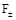being symmetric (+) or antisymmetric (-) under exchange of the two nucleons. The possible terms inobeying these conditions are products of spherical harmonics with spin triplet/singlet states, i.e.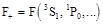and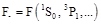resulting from the spin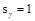of the absorbed photon and the properties of the deuteron ground state (The polar axis could be the direction of the incoming photon beam). Further details of the functions(see, e.g. ) play no role in the following conceptual discussion.

Before detection of a proton at a time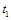, no possibility exists to differentiate between the two nucleons because their charges are indeterminate before and after the decay. Before the decay that is due to charge independence of the nuclear force and after the decay to the absence of any interaction of the two nucleons . Therefore the complete state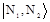of the outgoing two-nucleon system is a combination of spin-orbit states (9) and the isospin states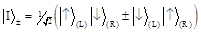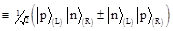(10)

where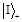is the entangled symmetric isospin triplet state and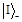the antisymmetric isospin singlet state of the np system. The statesand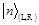represent the charge eigenstates of the nucleon, emitted into the left (L) or right (R) semi-space, respectively (Fig.2). One should note that before a detection the two charge eigenstates are not realized but only appear in the entangled states (10). The outgoing two-nucleon system generally is described according to the Pauli principle in the language of isospin (see, e.g., ) by the total antisymmetric state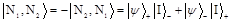(11)

where Ni (i = 1,2) denote the two nucleons. As long as no detection occurs, the nucleon charges are indeterminate because of the entangled states (10). Only a detection statistically occurring in 50% of the decay events records a proton: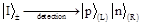(12)

That detection retroactively breaks the spherical symmetry of (9) by selection of a proton path (on a macroscopic scale) between the decay center and the detection point [10,11]. Thus a pre-existing proton is indicated and can be traced back to the deuteron decay center. It explains the quantum mechanical prediction (12) of the entangled state (10) identifying the second nucleon going into the right semi-space as neutron independent of its possibly space-like distance from the proton detection point and time.

On the other hand, if the detection is understood in the traditional way as an event without retroactive symmetry breaking (see, e.g. ), the undetected right-hand nucleon would remain with indefinite charge "knowing" nothing about its partner nucleon (detection or passage?). Only the assumption of a superluminal correlation or signal between the two nucleons violating Einstein locality could explain (12). That would be a paradoxical situation completely corresponding to Bohm’s version of EPR (reviewed in section (3). It always appears if pre-existing quantum numbers are not taken into account.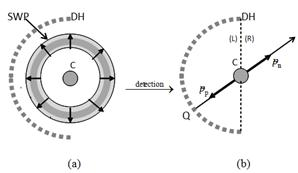Fig. 2. The left part (a) indicates the outgoing spherical wave packet SWP in plane projection describing particle motion between the decay center C and the detection hemisphere DH with indeterminate space angle, position and momentum. The right part (b) shows the situation after proton detection at the point Q. Now the two nucleon momenta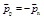a posteriori are determined by the detection.

5. Result and Discussion

In addition to Bohm’s version of the EPR arrangement, breaking of charge symmetry by proton detection after deuteron decay appears as an additional process of retroactive symmetry breaking giving more insight into the reason of the long-discussed seeming nonlocality problem in quantum mechanics.

The replacement of spin by isospin delivers an even more convincing argument favoring the acceptance of objective reality: In the example of section 4, the entangled isospin states (10) describe two outgoing nucleons with indefinite electric charge after deuteron decay. Because of our assumption that only charged particles can be detected, a detection at the left side of Fig. 2 only can be a proton detection that reduces the state (10) to (12) which identifies the other outgoing nucleon as neutron immediately after the proton detection. A corresponding coincidence measurement with minimally delayed neutron detection at the right side would confirm this. If one would assume (according to the conception of truth) that the proton detection is an event without retroactivity, one couldn’t understand the prediction (12) of the entangled state without a superluminal signal between the two detections.

On the other hand, dropping the conception of truth and accepting objective reality independent of observers, proton detection means that immediately before it a proton must have been very near the detection point and therefore must have been a proton also at former times preventing a nonlocality problem (see also ). Other than a pre-existing spin quantum number in (8), a pre-existing isospin quantum number concerns the identity of the particle itself and therefore is especially evident for experimentalists. Thus, the second nucleon leaving the decay center in opposite direction is identified as neutron without a superluminal signal.

As a general conclusion results: The conception of truth which forbids extrapolations of directly measured physical properties (quantum numbers) into regions without a possibility of direct measurement, leads to a contradiction between the well established theories of quantum mechanics and of special relativity and therefore should be eliminated.

References

1. J. S. Bell, Physics, vol. 1, 1965, p. 195.
2. S. J. Freedmann and J. F. Clauser, Phys. Rev. Lett., vol. 28, 1972, p. 938.
3. A.Einstein, B. Podolski and N. Rosen, Phys. Rev., vol. 47, 1935, p. 777.
4. D. Bohm, Quantum Theory, Prentice Hall: Englewood Cliffs, 1951, p. 614.
5. B. J. Hiley, Contemp. Phys., vol. 18, 1977, pp. 411-14.
6. H. Rauch, "More quantum information due to postselection in neutron interferometry",Fundamental Problems in Quantum Theory, Annals of the New York Academy of Sciences,vol. 755, 1994, p. 284.
7. R. Omnѐs, The Interpretation of Quantum Mechanics, Princeton University Press, 1994, p. 85.
8. N. D. Mermin, Pramana, vol. 51, 1998, p. 549.
9. R. B. Griffiths, 2003 Found. Phys., vol. 33, 2003, pp. 1423-25. 
10. K. Lewin, Found. Phys., vol. 39, 2009, pp. 1145-60.
11. K. Lewin, Quantum Mechanics, Series: Physics Research and Technology, Nova SciencePublishers, Inc., New York, 2011, pp. 25-28.
12. J. M. Blatt and V. F. Weisskopf, „Zweikörperprobleme bei niedrigen Energien", TheoretischeKernphysik, B. G. Teubner Verlagsgesellschaft, Leipzig 1959, pp. 41-82.
13. H. A. Bethe and F. de Hoffmann, Mesons and Fields, Row, Peterson and Company, WhitePlains, New York, vol. 2, 1957, p. 39.
14. I. Bloch, Phys. Rev., vol. 156, 1967, p. 1377.
15. K. Lewin, J. Phys., Conf. Ser., vol. 343, 2012, pp. 699-705.

Footnotes

The I-spin eigenvalues of a nucleon in a given z direction in I-spin space are  with Q =1  as proton charge, i.e.     for the proton  and  for the neutron .

At higher photon energies direct γN interactions during the decay process  become more important and would lead to different weight factors at the two terms of the entangled states (10) after the decay because of different electromagnetic properties of neutron and proton. In spite of that deviation from complete charge symmetry, the nucleon charges remain indeterminate before detection. That situation resembles the EPRB case where the passage of a fermion with indefinite spin through the inhomogeneous magnetic field of the Stern-Gerlach device leads to the two branch waves in (2).

 Contents 1. 2. 3. 4. 5.
Article ToolsAbstractPDF(220K)